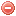# Description

The forest fire danger rating index ($$F$$) and fuel moisture index ($$FMI$$, a sub-index of $$F$$) were recently developed by Sharples et al. (2009a, 2009b). They require temperature, relative humidity and windspeed (for F) as input variables.

The purpose of these indices was to assess moisture content and fire danger in eucalypt forests in southern Australia in a very simple way and as effectively as with more sophisticated models (cf. $$FFDI$$ and $$FFWI$$).

The $$F$$ index is based on the principle that fire danger is determined by the combined influence of wind speed and fuel moisture content. It increases when wind speed increases and decreases when fuel moisture content increases (cf. equation below Sharples et al. 2009a).

NB: the $$FMI$$ is dimensionless and therefore is not a direct estimate of fuel moisture content (Sharples et al. 2009b).

# Formula

The $$FMI$$ is obtained as follows:

$FMI=10-0.25\cdot{(T-H)}$

where $$T$$ is air temperature and $$H$$ relative air humidity;

And $$F$$ as follows:

$F=\dfrac{max(U_0, U)}{FMI}$

where $$U$$ is wind speed in [km/h] and $$U_0$$ = 1 [km/h] (threshold value in order to ensure that that fire danger is always greater than 0, even when there is no wind).

The $$F$$ and $$FMI$$ indices are supposed to be calculated on a hourly basis. Thus the meteorological data used for its calculation are the meteorological data at the time of the basic weather observation.

In the case of the $$F$$ and of the $$FMI$$, no particular values or conditions are required when starting the index calculation.

# Modifications

The $$FMI$$ in the $$F$$ formula reflects short-term changes in fuel moisture contents. In order to take into account long-term moisture effects (or in other words fuel availability), Sharples et al. (2009a) proposed to include a drought factor in the F equation (a function of the $$KBDI_{SI}$$ (in millimeters); cf. $$FFDI$$). The modified $$F$$ formula would therefore be as follows:

$F_d=DF\cdot\dfrac{max(U_0, U)}{FMI}$

where $$DF$$ is the drought factor (cf. $$FFDI$$) defined as follows (Noble et al. 1980):

$DF=\dfrac{0.191\cdot{(KBDI+104)}\cdot{(w+1)^{1.5}}}{3.52w+1^{1.5}+P-1}$

where $$w$$ is the number of days since last rain and $$P$$ the last precipitation amount (measured over the entire period of rainfall).

NB: if the calculated drought factor is greater than 10, then $$DF$$ in the $$F_d$$ formula is equal to 10.

The $$F_d$$ index is aimed to be calculated on a hourly basis. Thus the meteorological data used for its calculation are the meteorological data at the time of the basic weather observation.

As the $$F_d$$ includes the $$KBDI_{SI}$$ in its formulation, the starting conditions of the $$KBDI_{SI}$$ have to be met, i.e. the soil layer has to be saturated with water, e.g. after a period of abundant rainfall, e.g. 152 or 203 mm in a period of a week. The $$KBDI_{SI}$$ component takes the starting value of 0.

Original publications:
Sharples et al. (2009a)
Sharples et al. (2009b)

Other publication:
Noble et al. (1980)

### Bibliography SearchNo results!

No results were found. Are you sure you searched for a tag?

### Symbols

 Variable Description Unit $$T$$ air temperature °C $$T_{dew}$$ dew point temperature °C $$H$$ air humidity % $$P$$ rainfall mm $$U$$ windspeed m/s $$w$$ days since last rain (or rain above threshold) d $$rr$$ days with consecutive rain d $$\Delta t$$ time increment d $$\Delta{e}$$ vapor pressure deficit kPa $$e_s$$ saturation vapor pressure kPa $$e_a$$ actual vapor pressure kPa $$p_{atm}$$ atmospheric pressure kPa $$PET$$ potential evapotranspiration mm/d $$r$$ soil water reserve mm $$r_s$$ surface water reserve mm $$EMC$$ equilibrium moisture content % $$DF$$ drought factor - $$N$$ daylight hours hr $$D$$ weighted 24-hr average moisture condition hr $$\omega$$ sunset hour angle rad $$\delta$$ solar declination rad $$\varphi$$ latitude rad $$Cc$$ cloud cover Okta $$J$$ day of the year (1..365/366) - $$I$$ heat index - $$R_n$$ net radiation MJ⋅m-2⋅d-1 $$R_a$$ daily extraterrestrial radiation MJ⋅m-2⋅d-1 $$R_s$$ solar radiation MJ⋅m-2⋅d-1 $$R_{so}$$ clear-sky solar radiation MJ⋅m-2⋅d-1 $$R_{ns}$$ net shortwave radiation MJ⋅m-2⋅d-1 $$R_{nl}$$ net longwave radiation MJ⋅m-2⋅d-1 $$\lambda$$ latent heat of vaporization MJ/kg $$z$$ elevation m a.s.l. $$d_r$$ inverse relative distance Earth-Sun - $$\alpha$$ albedo or canopy reflection coefficient - $$\Delta$$ slope of the saturation vapor pressure curve kPa/°C $$Cc$$ cloud cover eights $$ROS$$ rate of spread m/h $$RSF$$ rate of spread factor - $$WF$$ wind factor - $$WRF$$ water reserve factor - $$FH$$ false relative humidity - $$FAF$$ fuel availability factor - $$PC$$ phenological coefficient -

 Suffix Description $$-$$ mean / daily value $$_{max}$$ maximum value $$_{min}$$ minimum value $$_{12}$$ value at 12:00 $$_{13}$$ value at 13:00 $$_{15}$$ value at 15:00 $$_{m}$$ montly value $$_{y}$$ yearly value $$_{f/a}$$ value at fuel-atmosphere interface $$_{dur}$$ duration $$_{soil}$$ value at soil level

 Constant Description $$e$$ Euler's number $$\gamma$$ psychrometric constant $$G_{SC}$$ solar constant $$\sigma$$ Stefan-Bolzmann constant